Scattering of X-rays by 2- and 3-Dimensional Units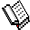Course Material Index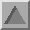Section Index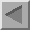Previous Page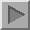Next Page

Scattering of X-rays by 2- and 3-Dimensional Units

We now move quickly up in dimension towards a 3D-crystal. Clearly the next case will be a two-dimensionally repeating set of scatterers as below:

2D crystal (a, b repeats) :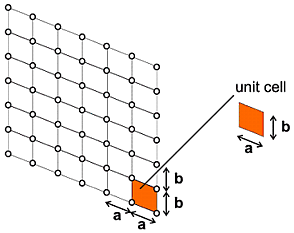von Laue condition S.a = h :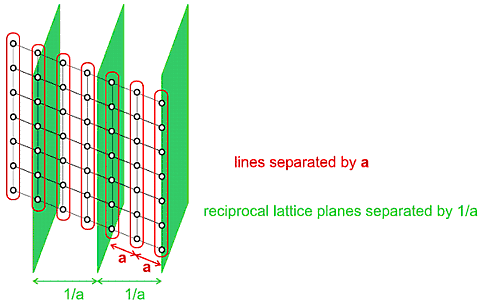von Laue condition S.b = k :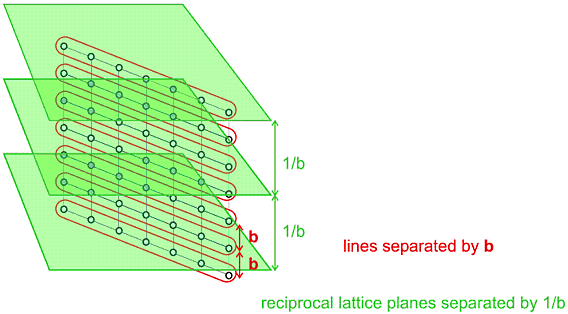The pattern repeats in two dimensions, the unit displacements being a and b respectively and the unit parallelogram formed (indicated) being known as the unit cell. The second version of the above diagram shows how we can re-visualise this so that atoms are grouped into rows of atoms spaced by a. From the arguments used in the previous section this would lead to a von Laue condition of a.S = h, which is equivalent to a set of parallel reciprocal lattice planes spaced by 1/a. However the third version of the above diagram indicates that we can alternatively visualise a different grouping of atoms, into rows spaced by b, which then leads to an alternative von Laue condition (b.S = k, say, an other integer) and an alternative set of parallel reciprocal lattice planes spaced by 1/b.

If then the 2-dimensional crystal leads to these two alternatives, we must say that both von Laue conditions must be simultaneously satisfied (i.e. two integers, say h and k, involved). Since diffraction will only occur when the reciprocal lattice planes intersect the Ewald sphere, yet we have two sets of reciprocal lattice planes begging this condition, then the only region where this "double condition" might be satisfied is where these two sets of planes intersect themselves and the Ewald sphere. Now two sets of parallel planes intersect as a set of parallel lines (see below) which themselves will intersect the Ewald sphere: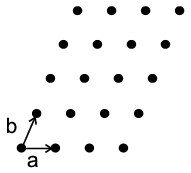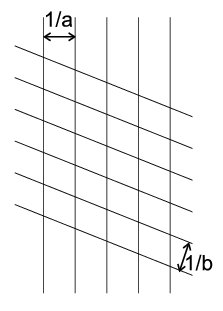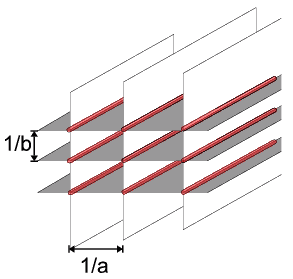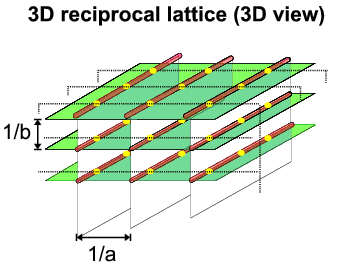The 3D-crystal is the next and last case; it gets somewhat difficult to draw though it is obvious that the above 2D arguments can be extended to 3D with the result that:
• in 3D, the unit cell is the solid parallelepiped formed by the sides a, b and c.
• 3 simultaneous von Laue conditions need to be satisfied:
• S.a = h, an integer
S.b = k, a second integer
S.c = l, a third integer
• the effective reciprocal lattice points must reside at the intersection of 3 sets of parallel planes. Three sets of parallel planes intersect as a lattice of points, known as reciprocal lattice points and shown above in yellow.
So there we have it: A three-dimensional crystal will diffract when the experimental geometry is such that one (or more) of its reciprocal lattice points intersects the Ewald sphere (radius 1/λ). The reciprocal lattice points will lie at the intersection of 3 sets of equidistant parallel planes which are angled perpendicular to the a-, b- and c- directions. For further explanation of the concepts of the Ewald sphere and the reciprocal lattice click here.Course Material IndexSection IndexPrevious PageNext Page
 © Copyright 1997-2006.  Birkbeck College, University of London. Author(s): Paul Barnes Simon Jacques Martin Vickers